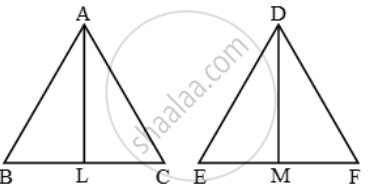# Two Isosceles Triangles Have Equal Vertical Angles and Their Areas Are in the Ratio 16 : 25. Find the Ratio of Their Corresponding Heights - Mathematics

Sum

Two isosceles triangles have equal vertical angles and their areas are in the ratio 16 : 25. Find the ratio of their corresponding heights

#### Solution

Let ∆ABC and ∆DEF be the given triangles such that AB = AC and DE = DF, ∠A = ∠D.

and \frac{Area\ (\Delta ABC)}{Area\ (\Delta DEF)}=\frac{16}{25}  …….(i)

Draw AL ⊥ BC and DM ⊥ EF.

Now, AB = AC, DE = DF

\Rightarrow \frac{AB}{AC}=1\text{ and }\frac{DE}{DF}=1\Rightarrow \frac{AB}{AC}=\frac{DE}{DF}\text{ }\Rightarrow \text{ }\frac{AB}{DE}=\frac{AC}{DF}

Thus, in triangles ABC and DEF, we have

\frac{AB}{DE}=\frac{AC}{DF}\text{ and } ∠A = ∠D [

So, by SAS-similarity criterion, we have

∆ABC ~ ∆DEF

\Rightarrow \frac{Area\ (\Delta ABC)}{Area\ (\DeltaDEF)}=(AL^2)/(DM^2)

\Rightarrow \frac{16}{25}=(AL^2)/(DM^2)

\frac{AL}{DM}=\frac{4}{5}

AL : DM = 4 : 5

Concept: Areas of Similar Triangles
Is there an error in this question or solution?